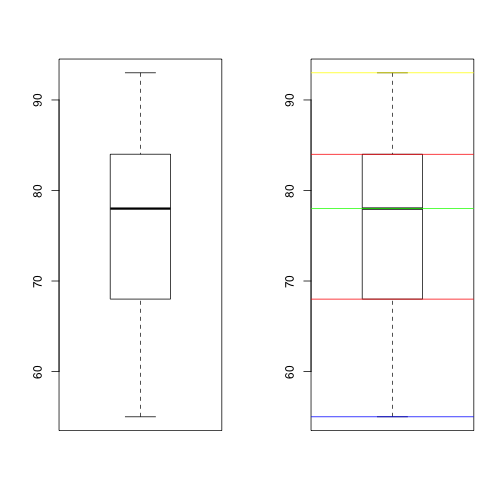# Data Analysis using R (Tutorial) - Five number summary statistics

Author : Abhinav Agrawal

Following, we will see how to pull the five point summary (Minimum, Maximum, Median, 1st Quartile, 2nd Quartile) statistics on a set of observations, and visualize the summary statistics using box plot.

For illustration purpose, lets just consider the test scores of 9 students in Physics.

``````scores <- c(78, 93, 68, 84, 90, 74, 64, 55, 80)  # Created the vector object scores using the c() function
scores  # print the scores
``````
``````##  78 93 68 84 90 74 64 55 80
``````

To begin with, let us go ahead and sort the scores,

``````sort(scores)
``````
``````##  55 64 68 74 78 80 84 90 93
``````

Just by seeing the sorted data, we can say that 55 is the minimum score and 93 is the maximum score.

We can also find the median. It is the score half way from the first to last observation. So, 1 + (9-1)/2 = 5, the score at 5th position is the median, which is 78.

(Median is the middle value of the observation which divides the data into 2 halves. Half of the observations have value less than median and half more than median).

In this case half of the scores are less than 78 and other half of the scores are more than 78.

Now, let us talk about quartiles. The 2 important quartiles are first quartile (or the lower quartile or 25% quartile) and the third quantile (upper quartile or 75% quartile)

The nth quartile of a sample data is the value that cuts off the first n percent of the data values when it is sorted in ascending order.

First quartile (25%) is one fourth way along from the first observation to the last observation. It divides the sample data in such a way that 25% of the values are less than the first quartile and 75% are more than first quartile.

Third Quartile (75%) is three fourth way along the way from the first observation to the last observation. It divides the sample data in such a way that 75% of the values are less than third quantile and 25% of the values are more than the third quantile.

Calculating the position of, First Quartile : ¼ the way along from the first value to the last value. We have 9 values. So, 1 + (9-1)/4 = 3rd position, 68 is the first quartile. Third Quartile : ¾ the way along from the first value to the last value. We have 9 values. So, 1 + (9-1)*¾ = 7th position, 84 is he thrid quartile.

This was easy as we just had 9 observations in our data set. However, when we work with large data sets, it would be easier to use the available R functions to get the summary statistics.

find minimum (lowest) score,

``````min(scores)
``````
``````##  55
``````

Maximum (highest) scores,

``````max(scores)
``````
``````##  93
``````

median of the scores,

``````median(scores)
``````
``````##  78
``````

finding the quartiles using the quantile () function.

``````quantile(scores)
``````
``````##   0%  25%  50%  75% 100%
##   55   68   78   84   93
``````

First Quartile or 25th quartile,

``````quantile(scores, 0.25)
``````
``````## 25%
##  68
``````

Third Quartile or 75th quartile,

``````quantile(scores, 0.75)
``````
``````## 75%
##  84
``````

25th, 50th and 75th quartile,

``````quantile(scores, c(0.25, 0.5, 0.75))
``````
``````## 25% 50% 75%
##  68  78  84
``````

All this information could easily be retrived using the summary() function on the object scores. Note that in addition to above 5 statistics, summary() function also gives the mean value.

``````fivenum(scores)
``````
``````##  55 68 78 84 93
``````
``````summary(scores)
``````
``````##    Min. 1st Qu.  Median    Mean 3rd Qu.    Max.
##    55.0    68.0    78.0    76.2    84.0    93.0
``````
``````par(mfrow = c(1, 2))
boxplot(scores)
boxplot(scores)
abline(h = min(scores), col = "Blue")
abline(h = max(scores), col = "Yellow")
abline(h = median(scores), col = "Green")
abline(h = quantile(scores, c(0.25, 0.75)), col = "Red")
``````